# Fourier coefficients of automorphic forms

Jump to: navigation, search

Many of the applications of automorphic forms (cf. also Automorphic form) involve their Fourier coefficients. Here, the special case of holomorphic modular formsof weightfor the full modular group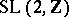will be considered. Ifis a such a modular form, then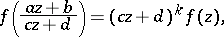for all integers,,,such that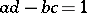andin the upper half-plane. Therefore it has periodin the real part ofand must have a Fourier expansionThe numbersare the Fourier coefficients of. The modular form is a cusp form if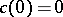. Some references are [a5], [a6], [a7], [a10], [a11], [a12], [a15] (see also the extensive bibliography in this).

For Eisenstein series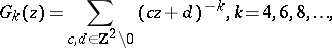the non-zero Fourier coefficients are essentially divisor functionsThe theta-function associated to a positive-definite real symmetric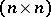-matrixand a pointin the upper half-plane is defined by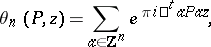where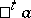denotes the transpose of the column vector. One says thatis an even integral matrix when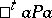is an even integer, for all integral column vectors. In this case,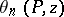is a modular form of weight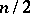. Then theth Fourier coefficient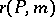ofis the number of representations ofas, for some integer vector. It follows from the one-dimensionality of the space of modular forms of weightthat when,where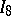denotes the identity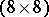-matrix.

There are many more examples of number-theoretically interesting Fourier coefficients. The Fourier coefficients of the inverse of the Dedekind eta-function involve the partition function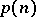, which is the number of ways of writingas a sum of positive integers. The discriminant function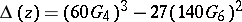is a cusp form whose Fourier coefficients are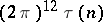, where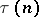is the Ramanujan tau-function (cf. also Ramanujan function). S. Ramanujan conjectured thatwhenandare relatively prime. This was proved by L.J. Mordell. E. Hecke created the theory of Hecke operators, which gave a general framework for such results. Ramanujan also conjectured that for all prime numbersone has the inequality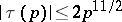. P. Deligne proved this conjecture as a special case of a much more general result holding for all cusp forms. This result has applications in extremal graph theory (cf. also Graph theory); [a7], [a10].

Two other conjectures are still (1998) open. Computations lead one to believe that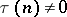, for all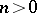. Writing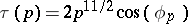, J.-P. Serre conjectured that the angles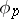are distributed in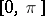with respect to the measure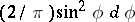(the Sato–Tate or Wigner semi-circle distribution).

The modular invariant is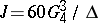. The functionhas integer Fourier coefficients (writing)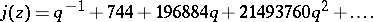There is a surprising connection of these Fourier coefficients with the representations of the largest of the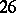sporadic finite simple groups (the Monster; cf. also Simple finite group). All of the early Fourier coefficients ofare simple linear combinations of degrees of characters of the Monster group. This led J.H. Conway [a1] to refer to the "moonshine" properties of the Monster. In 1998, R.E. Borcherds won the Fields Medal in part because he proved the Conway–Norton "moonshine conjecture" ; see [a17].

The Hecke correspondence maps a modular formwith Fourier coefficients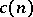to an-function (cf. also Dirichlet-function) given by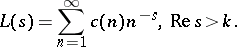This-function has an analytic continuation and a functional equation. The Riemann zeta-function corresponds to the simplest theta-function. But here the weight of the modular form isandis not invariant under the full modular group.

Ifis a form of integer weight for the modular group which is an eigenfunction for all the Hecke operators, then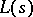has an Euler product. There are also converse theorems. The result is that many of the important zeta- and-functions of number theory correspond to modular forms.

The Taniyama–Shimura–Weil conjecture says that the zeta-function of an elliptic curve over the rational numbers should come via the Hecke correspondence from a cusp form of weight(for a congruence subgroup of the modular group). This conjecture is intrinsically linked with the proof by A. Wiles [a16] of Fermat's last theorem (cf. also Fermat last theorem). In 1999, B. Conrad, F. Diamond and R. Taylor proved the Taniyama–Shimura–Weil conjecture when the conductor is not divisible by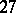(see [a18]) and announced with C. Breuil a proof of the conjecture in general.

Maass wave forms (see [a8]) are eigenfunctions of the non-Euclidean Laplacian on the upper half-plane which are invariant under the modular group. Their Fourier expansions involve-Bessel functions and the Fourier coefficients are more mysterious, except for those of the Eisenstein series, which are still divisor functions. Computer studies have been carried out in, e.g., [a4], [a14]. The analogue of the Ramanujan conjecture is still (1998) open.

Siegel modular forms are holomorphic functions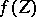, whereis a complex symmetric-matrix with positive-definite imaginary part, such thatfor all matrices,,,with integer entries and such that with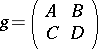one has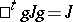, wherewith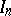the identity-matrix. Thenhas a Fourier expansionHere, "semi-integral" means that the diagonal entries ofare integers while the off-diagonal entries are either integers or halves of integers. Examples of Siegel modular forms include Eisenstein series and theta-functions. The Siegel main theorem on quadratic forms is a consequence of a formula relating the two. The analogue of the Hecke correspondence can be discussed. See [a13], for applications to the theory of quadratic forms, or [a9].

When looking at modular forms for groups with more than one cusp, such as congruence subgroups of the modular group or Hilbert modular forms for, with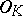the ring of integers of a totally real algebraic number field, there will be Fourier expansions at each cusp. Once more, Eisenstein series give examples. It is also possible to consider modular forms over imaginary quadratic number fields or even arbitrary number fields. See [a2]. The adelic version of the theory can be found in [a3] and [a7], for example.